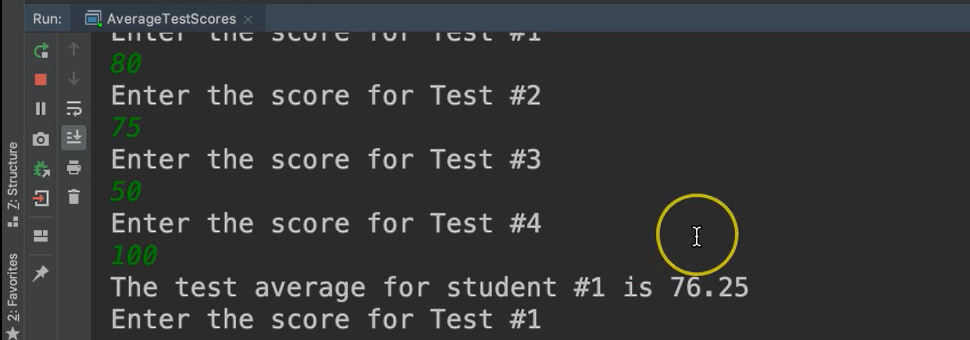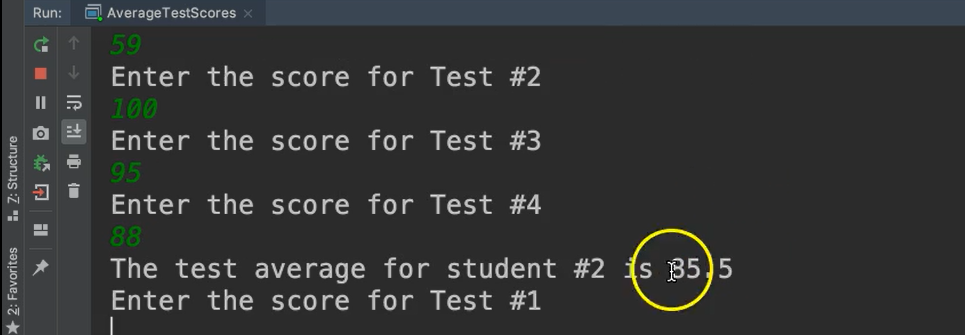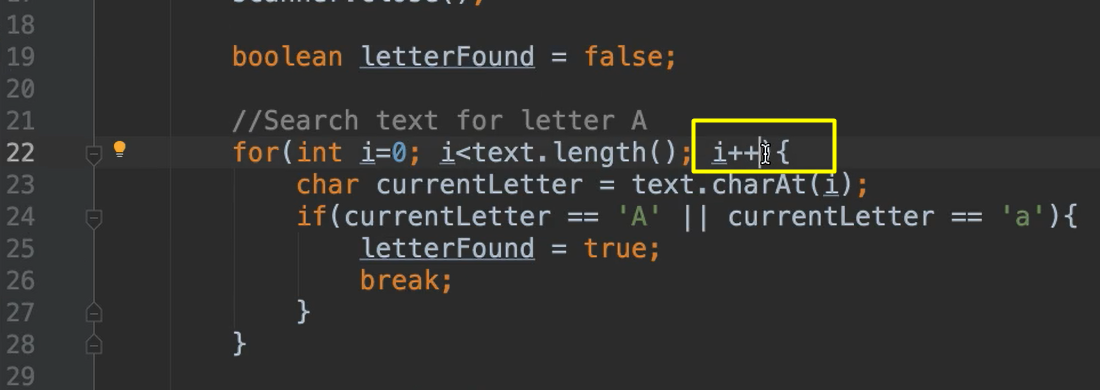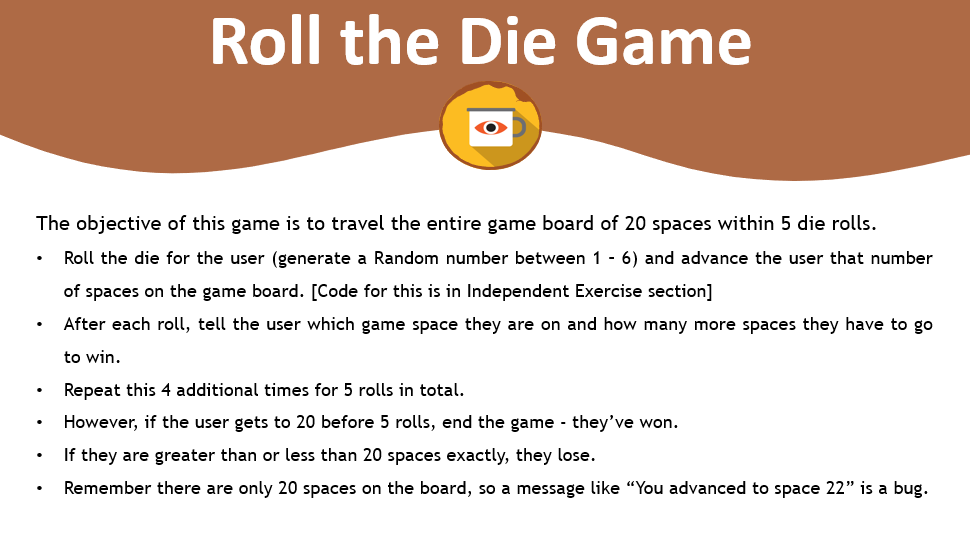Transcripted Summary

Sometimes your repetitive tasks also contain repetitive sub-tasks. In the case, you need a loop inside of a loop.

These are also known as nested loops.

Let's code an example where we need to find the average test scores for each student in the class.I went ahead and initialized the things that we know.

``````/*
* NESTED LOOPS:
* Find the average of each student's test scores
*/
public class AverageTestScores {

public static void main(String args[]){

//Initialize what we know
int numberOfStudents = 24;
int numberOfTests = 4;
}
}
``````

We know that there are 24 students in the class and that there's 4 tests that have been given.

Now we need to go through all of the students, all 24 of them. Since we know how many there are, we can use the `for` loop.

``````//Process all students
for(int i=0; i< numberOfStudents; i++){

}
``````

Each iteration of this loop will focus on one student. Now that we have an individual student, we need to look at all 4 of their tests, which means we're going to need another loop.

We also know the count of that, so this would be a `for` loop as well.

As we're making this `for` loop we have to be careful — you cannot use the same sentinel `i` because this sentinel will still be valid as we're inside of this second loop.

So, we have to use a totally different sentinel `j`, which will control this inner `for` loop.

As we go inside of this inner `for` loop, we're going to ask the user to give us the test score and then we're going to add that test score to an accumulating variable called `total`.

``````//Process all students
for(int i=0; i< numberOfStudents; i++){

double total = 0;
for(int j=0; j<numberOfTests; j++){

}
}
``````

Because we need to add this to `total` each time, we're going to need to declare `total` before we get inside of the loop. Then we can update total inside of here. If we would have put `total` inside of the inner loop, it would have been overwritten for every iteration and it wouldn't accumulate.

Now we can prompt the user asking them to enter the score and then add it to this total.

Let's do that.

``````for(int i=0; i< numberOfStudents; i++){

double total = 0;
for(int j=0; j<numberOfTests; j++){
System.out.println("Enter the score for Test #" + (j+1));
double score = scanner.nextDouble();
total = total + score;
}
}
``````

In the print statement, I just entered this `j + 1` because I want to use this number within the String. So, this is not doing anything to the `j` variable, it's not incremented or anything. This is simply for display.

This will say "Enter the score for Test #1" and the reason there's this plus one is because `j` is 0 right now — so 0 + 1 will be 1 for the first one and so on.

So, now we've gotten the score.

We're going to run through this 4 times for student and we'll have their final total here.

After we finish this inner loop, we're still on the same student because we're still inside of here. We'll go ahead and get their test average and then we'll print it out.

``````//Process all students
for(int i=0; i< numberOfStudents; i++){

double total = 0;
for(int j=0; j<numberOfTests; j++){
System.out.println("Enter the score for Test #" + (j+1));
double score = scanner.nextDouble();
total = total + score;
}

double average = total/numberOfTests;
System.out.println("The test average for student #" + (i+1) + " is " + average);
}
``````

Okay, let's take a look at what we have here.

### # AverageTestScores.java

``````package chapter4;

import java.util.Scanner;

/*
* NESTED LOOPS:
* Find the average of each student's test scores
*/
public class AverageTestScores {

public static void main(String args[]){

//Initialize what we know
int numberOfStudents = 24;
int numberOfTests = 4;

Scanner scanner = new Scanner(System.in);

//Process all students
for(int i=0; i< numberOfStudents; i++){

double total = 0;
for(int j=0; j<numberOfTests; j++){
System.out.println("Enter the score for Test #" + (j+1));
double score = scanner.nextDouble();
total = total + score;
}

double average = total/numberOfTests;
System.out.println("The test average for student #" + (i+1) + " is " + average);
}

scanner.close();
}
}
``````

We have the initial `for` loop. This will go through each student.

Let's say I'm starting off with the first student. Their total is 0 because I haven't counted anything yet.

Let me look through their 4 tests. I'm going to look at the first test, I'm going to ask them the score of that. Then I'm going to read it in and then add it to their total. At first, it was 0, but whatever they put in will be added to that.

Then it's going to come back to the top of this loop because we're not done with it yet. We have to continue with this loop until we get to the number of tests. I'll go and ask for test number 2. Add that to the total. Do the same for test 3, same for test 4.

Once it completes this loop is when it will exit the inner `for` loop.

But we're still within this outer loop, so we'll say the average is the total divided by the number of tests. Now that we have the average we can go ahead and print. We're still on student 1 and their average.

Once this is complete, it will come back to the top of the outer loop, then go to the next student and do the same thing.

Let's run it.

We'll say their first score is an 80, then they got a 75 and then they got 50 and then they really cleaned up their act and got 100.Let's see, the test average for student number #1 is 76.

Then it continues on. You go and ask for a test again.

We'll just do one more student so you can see that it's #2 — 59, 100, 95 and then 88.See student 2 has an 85.5. This will continue on for all 24 students.

There's a couple more things that I want to highlight.

Let's go to the LetterSearch program from Chapter 4.3 again.Let’s talk about this incrementer. We've seen examples where we use the `++`.

We could also use a `--`. Let's say that we wanted to traverse this String from the last character to the first character.

We would change this up:

• `i` would be begin at the last letter, which can be found with `text.length()`
• the condition would be while `i > 0`
• then we could use `i--`
``````for(int i=text.length(); i>0; i--){

}
``````

Also, this middle statement, the condition, does not have to be limited to just this sentinel `i`.

We can add any condition here or we can even use compound conditions. For example, we used the `break` to say if we find the 'A', go ahead and break out of this. This is one option.

The beautiful thing about programming is that you can implement things in multiple ways. Instead of using the `break` statement, we could leave this where we update `letterFound` to `true` and say to continue looping while the letter is not found, and we don't have anymore characters to go through.

We could change this condition in that way.

``````//Search text for letter A
for(int i=0; !letterFound && i<text.length(); i++){
char currentLetter = text.charAt(i);
if(currentLetter == 'A' || currentLetter == 'a'){
letterFound = true;
}
}
``````

## Optional Independent ExerciseThe objective of this game is to travel the entire game board of 20 spaces within 5 die rolls.

• Roll the die for the user (generate a Random number between 1 – 6) and advance the user that number of spaces on the game board. Here’s the code to do this ((import is java.util.Random):

``````Random random = new Random();
int die = random.nextInt(6) + 1;
``````
• After each roll, tell the user which game space they are on and how many more spaces they have to go to win.

• Repeat this 4 additional times, for 5 rolls in total.

• However, if the user gets to 20 before 5 rolls, end the game - they’ve won.

• If they are greater than or less than 20 spaces exactly, they lose.

• Remember there are only 20 spaces on the board, so a message like “You advanced to space 22” is a bug.

Example Output

``````Roll #1: You've rolled a 3. You are now on space 3 and have 17 more to go.

Roll #2: You've rolled a 3. You are now on space 6 and have 14 more to go.

Roll #3: You've rolled a 3. You are now on space 9 and have 11 more to go.

Roll #4: You've rolled a 5. You are now on space 14 and have 6 more to go.

Roll #5: You've rolled a 6. You're on space 20. Congrats, you win!
``````

Solution

Programming can be done many different ways, but here’s my solution.